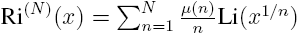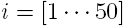# Improving Riemann prime counting

Michel Planat and Patrick Solé
Notes on Number Theory and Discrete Mathematics, ISSN 1310-5132
Volume 21, 2015, Number 3, Pages 38—44

## Details

### Authors and affiliations

Michel PlanatInstitut FEMTO-ST, CNRS,
15 B Avenue des Montboucons, F-25044 Besançon, France

Patrick SoléTelecom ParisTech, 46 rue Barrault, 75634 Paris Cedex 13, France.
Mathematics Department, King Abdulaziz University,
Jeddah, Saudi Arabia

### Abstract

Prime number theorem asserts that (at large x) the prime counting function π(x) is approximately the logarithmic integral li(x). In the intermediate range, Riemann prime counting functiondeviates from π(x) by the asymptotically vanishing sum ΣρRi(xρ) depending on the critical zeros ρ of the Riemann zeta function ζ(s). We find a fit π(x) ≈ Ri(3){ψ(x)} (with three to four new exact digits compared to li(x)) by making use of the Von Mangoldt explicit formula for the Chebyshev function ψ(x). Another equivalent fit makes use of the Gram formula with the variable ψ(x). Doing so, we evaluate π(x) in the range x = 10i,with the help of the first 2×106 Riemann zeros ρ. A few remarks related to Riemann hypothesis (RH) are given in this context.

### Keywords

• Prime counting
• Chebyshev psi function
• Riemann hypothesis

### AMS Classification

• Primary 11N05
• Secondary 11A25, 11N37

### References

1. Edwards H. M. (1974) Riemann’s zeta function, Academic Press, New York.
2. Odlyzko, A. Tables of zeros of the Riemann zeta function, available at http://www.dtc.umn.edu/~odlyzko/zeta_tables/.
3. Planat, M., & Solé, P. (2013) Efficient prime counting and the Chebyshev primes, J. Discrete Math. (Hindawi), Article ID 491627, 11 pp.
4. Borwein, J. B., Bradley, D. M., & Crandall, R. E. (2000) Computational strategies for the Riemann zeta function, J. Comp. Appl. Math., 121, 247–296.
5. Robin, G. (1984) Sur la difference Li(θ(x)) − π(x), Ann. Fac. Sc. Toulouse, 6, 257–268.
6. Pletser, V. Conjecture on the value of π(1026), the number of primes < 1026, Preprint 1307.4444 [math.NT].
7. Davenport, H. (1980) Multiplicative number theory, Second edition, Springer Verlag, New York.
8. Skewes, S. (1933) On the difference π(x) − li(x), J. London Math. Soc., 8, 277–283.
9. Burnol, J. F. The explicit formula in simple terms, Preprint 9810169 (math.NT).
10. Deléglise, M., & Rivat, J. (1998) Computing ψ(x), Math. Comp. 67, 1691–1696.
11. Alamadhi, A., Planat, M. & Solé, P. (2013) Chebyshev’s bias and generalized Riemann hypothesis, J. Alg., Numb Th.: Adv. and Appl., 8, 41–55; Preprint 1112.2398 (math.NT).

## Cite this paper

APA

Planat, M. & Solé, P. (2015). Improving Riemann prime counting. Notes on Number Theory and Discrete Mathematics, 21(3), 38-44.

Chicago

Planat, Michel, and Patrick Solé. “Improving Riemann Prime Counting.” Notes on Number Theory and Discrete Mathematics 21, no. 3 (2015): 38-44.

MLA

Planat, Michel, and Patrick Solé. “Improving Riemann Prime Counting.” Notes on Number Theory and Discrete Mathematics 21.3 (2015): 38-44. Print.